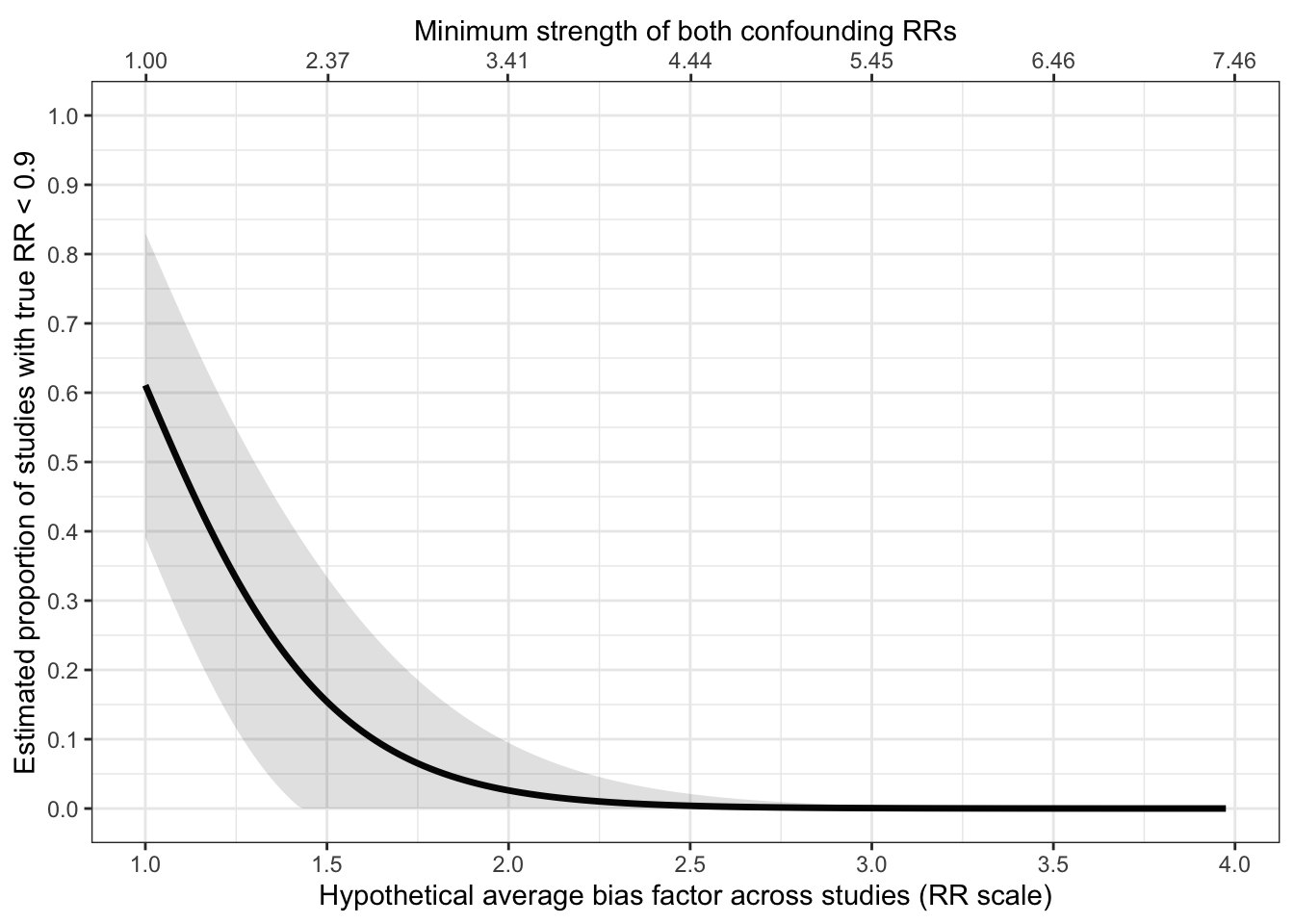## Example from Sensitivity Analysis for Unmeasured Confounding in Meta-Analyses

Trock et al. (2006)1 conducted a meta-analysis of observational studies (12 case-control and 6 cohort or nested case-control) on the association of soy-food intake with breast cancer risk. First we will meta-analyze the studies:

data(soyMeta)
( m = rma.uni(yi = soyMeta$est, vi = soyMeta$var,
method = "PM",
test = "knha") )
#>
#> Random-Effects Model (k = 20; tau^2 estimator: PM)
#>
#> tau^2 (estimated amount of total heterogeneity): 0.0973 (SE = 0.0505)
#> tau (square root of estimated tau^2 value):      0.3119
#> I^2 (total heterogeneity / total variability):   72.92%
#> H^2 (total variability / sampling variability):  3.69
#>
#> Test for Heterogeneity:
#> Q(df = 19) = 51.8693, p-val < .0001
#>
#> Model Results:
#>
#> estimate      se     tval  df    pval    ci.lb    ci.ub
#>  -0.1931  0.0882  -2.1899  19  0.0412  -0.3777  -0.0085  *
#>
#> ---
#> Signif. codes:  0 '***' 0.001 '**' 0.01 '*' 0.05 '.' 0.1 ' ' 1
yr = as.numeric(m$b) # returned estimate is on log scale vyr = as.numeric(m$vb)
t2 = m$tau2 vt2 = m$se.tau2^2 

Now, following Mathur & VanderWeele (2019)2, we will parametrically estimate the proportion of meaningfully strong protective effects (which we chose to define in this context as $$RR<0.90$$). First we will do so without correcting for confounding by specifying $$\texttt{muB} = 0$$ and $$\texttt{sigB} = 0$$.3

( res0 = confounded_meta(method = "parametric",
q = log(0.9),
tail = "below",
muB = 0,
sigB = 0,
yr = yr,
vyr = vyr,
t2 = t2,
vt2 = vt2) )
#> Cannot compute Tmin or Gmin without r. Returning only prop.
#>   Value       Est        SE    CI.lo     CI.hi
#> 1  Prop 0.6107838 0.1119729 0.391321 0.8302467
#> 2  Tmin        NA        NA       NA        NA
#> 3  Gmin        NA        NA       NA        NA

$$\texttt{Prop}$$ tells us that, prior to any correction for confounding, we estimate that 61% (95% CI: [39%, 83%]) of effects are meaningfully protective by the criterion $$RR<0.90$$.

Now let’s introduce hypothetical confounding bias that is log-normal across studies. In particular, let’s suppose that studies’ relative risks are biased away from the null by a factor of 1.3 on average (i.e., $$\texttt{muB} = \log{(1.3)}$$), and that these “bias factors” vary across studies such that 50% of the apparent effect heterogeneity across studies ($$\widehat{\tau}^2$$) was actually due to variation in confounding severity (i.e., $$\texttt{sigB} = \sqrt{\widehat{\tau}^2 / 2} =$$ 0.22). We will consider bias that on average shifts studies’ estimates away from the null, which is the usual choice in sensitivity analyses and is the default in $$\texttt{confounded_meta}$$. With the same function call, we will also estimate the severity of confounding that would be required to reduce to less than 10% ($$r=0.10$$) the proportion of studies with meaningfully protective true causal effects.

( res1 = confounded_meta(method = "parametric",
q = log(0.9),
tail = "below",
r = 0.10,
muB = log(1.3),
sigB = 0.2,
yr = yr,
vyr = vyr,
t2 = t2,
vt2 = vt2) )
#>   Value       Est        SE    CI.lo     CI.hi
#> 1  Prop 0.2328057 0.1494809 0.000000 0.5257828
#> 2  Tmin 1.4835598 0.2393508 1.014441 1.9526787
#> 3  Gmin 2.3305485 0.5172952 1.316669 3.3444285

$$\texttt{Prop}$$ tells us that, under the severity and variability of confounding bias described above, the percentage of meaningfully protective effects would be reduced from our previous naive estimate of 61% to 23% (95% CI: [0%, 53%]). We no longer have a majority of meaningfully strong effects, but we do have a non-negligible minority (but the confidence interval indicates considerable uncertainty). $$\texttt{Tmin}$$ tells us that, when considering confounding of homogeneous strength across studies, we estimate that if each study’s relative risk were biased away from the null by a factor of 1.48 (95% CI: [1.01, 1.95]), this would be sufficiently severe confounding to reduce to less than 10% the percentage of meaningfully strong true causal effects. We can also express this statement in terms of confounding strength: $$\texttt{Gmin}$$ tells us that, if each study had confounder(s) that were associated with both soy intake and with breast cancer risk by relative risks of at least 2.33-fold (95% CI: [1.32-fold, 3.34]) each, then this could be sufficiently severe confounding to reduce to less than 10% the percentage of meaningfully strong true causal effects.4 We can plot the hypothetical severity of bias (here treated as homogeneous across studies, i.e., $$\texttt{sigB}=0$$) versus the estimated proportion of meaningfully strong effects as follows:

sens_plot(method = "parametric",
type = "line",
q = log(0.9),
sigB = 0,
tail = "below",

yr = yr,
vyr = vyr,
t2 = t2,
vt2 = vt2)
#> Warning in sens_plot(method = "parametric", type = "line", q = log(0.9), :
#> Calculating parametric confidence intervals in the plot. For values of the
#> proportion that are less than 0.15 or greater than 0.85, these confidence
#> intervals may not perform well.The warning message reminds us that it is better to use the calibrated method when the proportion is extreme. This method also avoids assuming that the population effects are normal across studies, as we did when using the parametric method. However, the calibrated method only considers bias that is homogeneous across studies. Here is what the plot looks like when using the calibrated method:

sens_plot(method = "calibrated",
type = "line",
q = log(0.9),
tail = "below",
sigB = 0,
dat = soyMeta,
yi.name = "est",
vi.name = "var",
give.CI = FALSE)